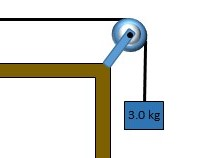# Tension is maintained in a string as in the figure. The observed wave speed is v= 20 m/s when the...

## Question:

Tension is maintained in a string as in the figure. The observed wave speed is v= 20 m/s when the suspended mass is m= 3.0 kg.

Find the frequency if the string is 0.5 m long.## Wave Parameters:

The wave can be characterized by its wavelength, or the distance in space between consecutive crests or troughs, the speed with which the wave propagates, which depends on the medium the wave propagates in, and the frequency, or the number of times per second the amplitude is reached at a given point in the space.

## Answer and Explanation:

The wave speed is defined as follows:

v = \lambda f

#### Learn more about this topic:Wave Parameters: Wavelength, Amplitude, Period, Frequency & Speed

from CLEP Natural Sciences: Study Guide & Test Prep

Chapter 8 / Lesson 2
324K